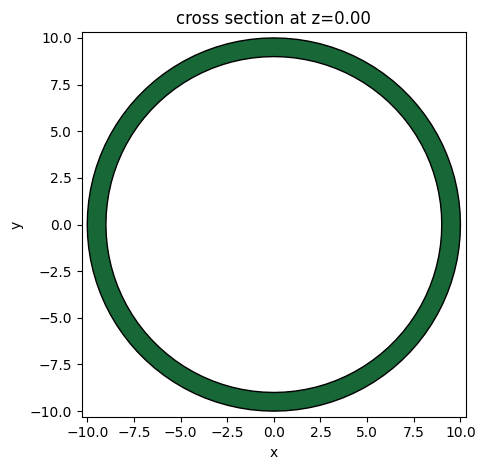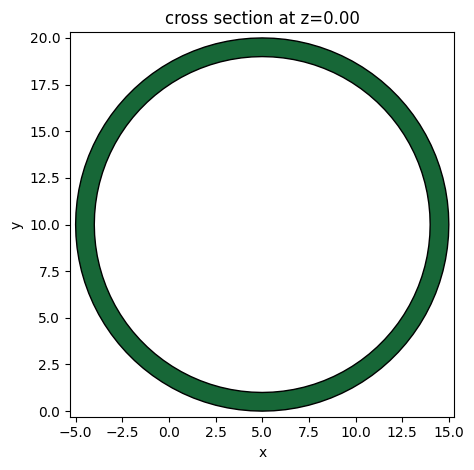# Defining complex geometries using trimesh#

Tidy3D offers four primitive geometric shapes: Box, Cylinder, Sphere, and PolySlab. An extensive array of intricate geometrical configurations can be defined from these fundamental building blocks by manipulating their properties and hierarchical arrangements. For instance, our tutorials on the Luneburg lens waveguide size converter and the Fresnel lens showcase the creation of curved surfaces through the strategic layering of cylindrical elements.

Beyond the in-built primitives, Tidy3D accommodates external geometrical specifications in either the gds or stl file formats, allowing users to import custom geometries created in their preferred design tools. Additionally, compatibility with external libraries like gdstk and shapely opens the gateway to even more complex geometric possibilities. Using gdstk to create various waveguide structures has been demonstrated in various examples such as the polarization splitter and rotator based on 90 degree bends.

In this tutorial, we’ll introduce an alternative avenue for crafting complex geometries using the trimesh library. Through examples featuring a torus and an ellipsoid, we’ll guide you through the process of defining a mesh structure that is directly usable in Tidy3D simulations.

Remember to install Tidy3D as pip install "tidy3d[trimesh]", which will install optional dependencies needed for processing surface meshes.

:

import tidy3d as td
import trimesh
import numpy as np
import matplotlib.pyplot as plt


## Built-in Geometries#

First off all, trimesh provides some built-in geometries such as ring (annulus), box, capsule, cone, cylinder, and so on. Let’s create a ring as an example.

After the mesh is created, we can visualize the mesh in 3D.

:

n_sections = 100 # how many sections to discretize the mesh

# create a ring mesh
ring_mesh = trimesh.creation.annulus(r_min=9, r_max=10, height=1, sections=n_sections)

# plot the mesh
ring_mesh.show()

:


To use this geometry in a Tidy3D simulation, we need to convert the mesh into a Tidy3D TriangleMesh geometry. The from_trimesh() method conviently converts a mesh to a Tidy3D geometry. From there, you can further define Tidy3D Structure and put it into a Simulation.

:

# define a tidy3d geometry from a mesh
ring_geo = td.TriangleMesh.from_trimesh(ring_mesh)

# plot a 2d cross section of the geometry
ring_geo.plot(z=0)
plt.show()

[10:57:15] WARNING: Plotting a 'TriangleMesh' may give inconsistent  mesh.py:415
results if the mesh is not unionized. We recommend
unionizing all meshes before import. A
'PermittivityMonitor' can be used to check that the meshIn addition, you can easily apply operations to a mesh such as translation and rotation. Here we demonstrate how to translate the previously created ring by a vector (5, 10, 0). The resulting ring is centered at (5, 10, 0).

:

# translate the ring
ring_mesh.apply_translation([5,10,0])

# define a tidy3d geometry from a mesh
ring_geo = td.TriangleMesh.from_trimesh(ring_mesh)
ring_geo.plot(z=0)
plt.show()

[10:57:16] WARNING: Plotting a 'TriangleMesh' may give inconsistent  mesh.py:415
results if the mesh is not unionized. We recommend
unionizing all meshes before import. A
'PermittivityMonitor' can be used to check that the meshIn a grating coupler simulation, very often we want to use a tilted cylinder to model the fiber core at an angle. This can be done with the help of trimesh too. First create a cylinder and then apply a rotation transformation to it.

:

# create a cylinder

: